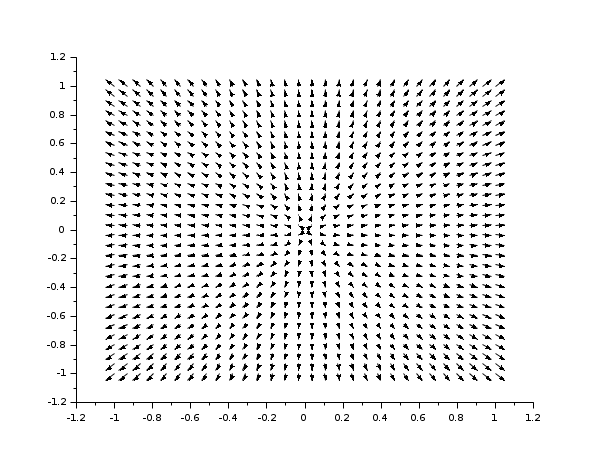Scilab Home page | Wiki | Bug tracker | Forge | Mailing list archives | ATOMS | File exchange
Change language to: English - Português - 日本語 - Русский

See the recommended documentation of this function

Aide de Scilab >> Graphiques > 2d_plot > champ

# champ

2D vector field plot

### Calling Sequence

```champ(x, y, fx, fy, [arfact, rect, strf])
champ(x, y, fx, fy, <opt_args>)```

### Arguments

x, y

two vectors which define the grid.

fx

a matrix which describes the x component of the vector field. `fx(i,j)` is the x component of the vector field at point `(x(i),y(j))`.

fy

a matrix which describes the y component of the vector field. `fy(i,j)` is the y component of the vector field at point `(x(i),y(j))`.

<opt_args>

This represents a sequence of statements `key1=value1, key2=value2`,... where `key1`, `key2`,... can be one of the following: `arfact`, `rect`, `strf` (see below).

arfact

an optional argument of type `real` which gives a scale factor for the display of the arrow heads on the plot (default value is `1.0`).

rect

a vector `rect=[xmin, ymin, xmax, ymax]` which gives the boundaries of the graphics frame to use.

strf

a string of length 3 "xyz" which has the same meaning as the `strf` parameter of `plot2d`. The first character `x` has no effect with `champ`.

### Description

`champ` draws a 2D vector field. The length of the arrows is proportional to the intensity of the field.

If you want colored arrows with the color of the arrows depending on the intensity of the field, use `champ1`.

Enter the command `champ()` to see a demo.

Be cautioned about the fact that using meshgrid function before using `champ` requires adjustment. For more details, see the last example below.

### Examples

```// using rect as plot boundaries
champ(-5:5,-5:5,rand(11,11),rand(11,11),rect=[-10,-10,10,10],arfact=2)``````// using (x,y) to get boundaries
clf()
champ(-5:5,-5:5,rand(11,11),rand(11,11),2,[-10,-10,10,10],"021")``````// using meshgrid to get fx and fy
clf()
x = linspace(-1,1,30);
y = linspace(-1,1,30);
[X,Y] = meshgrid(x,y);
fy = 2.*Y;
fx = 2.*X;
champ(x,y,fx',fy'); //here we have transposed fx and fy```• champ1 — 2D vector field plot with colored arrows
• fchamp — direction field of a 2D first order ODE
• plot2d — 2D plot